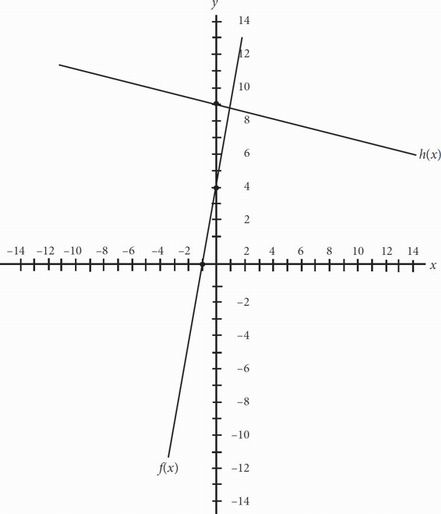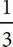# SAT Math Multiple Choice Question 137: Answer and Explanation

### Test Information

Question: 137

2.Two graphs, f(x) and h(x), are shown above. If f(x) = 3x + 4 and f(x) and h(x) are perpendicular, which of the following could be the equation of h(x) ?

• A. h(x) =x + 9
• B. h(x) = -x + 9
• C. h(x) = 3x + 9
• D. h(x) = -3x + 9

BDon't get too thrown off by the graph. All you need to know to solve this question is that perpendicular lines have slopes that are the negative reciprocals of each other. Since the standard equation for a line is y = mx + b, the slope of the f(x) line is 3. The slope of the h(x) line must therefore be -. The only answer choice that matches is (B).